# Lines of Symmetry

Lines of Symmetry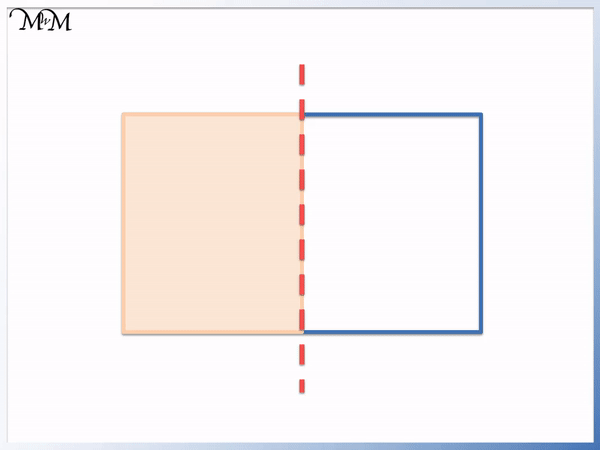• A line of symmetry is a line that cuts a shape in half.
• The shape will look the same on both sides of the line of symmetry.
• We can mark lines of symmetry using a dashed line drawn on the shape.
• We can find lines of symmetry using a mirror. We have a line of symmetry if the reflection of the shape in the mirror looks the same as the shape in front of the mirror.• A line of symmetry can be found by folding the shape.
• If the shape can be folded in half, the line of the fold is a line of symmetry.
• We fold the shape over and if one half lies exactly on top of the other half, we have a line of symmetry.

Lines of symmetry are lines that divide shapes in half.

Both sides of the line of symmetry look exactly the same.• A square has 4 different lines of symmetry: one on each diagonal, one vertical line and one horizontal line.
• A regular shape is a shape with sides of the same length. All regular shapes have the same number of lines of symmetry as they do sides.Supporting Lessons# Lines of Symmetry

## What are Lines of Symmetry?

A line of symmetry is a line that divides a shape exactly in half. They are drawn with a dashed line. Lines of symmetry can be found by folding a shape in half or using a mirror. Each side of the line of symmetry will look exactly the same. For example, a square has 4 lines of symmetry.Lines of symmetry look like dashed lines. The shape on each side of the line looks exactly the same.

## How to Find Lines of Symmetry

To find a line of symmetry use any of these tests:

1. If both sides of a shape look exactly the same when it is folded, this fold line is a line of symmetry.
2. If the reflection of the shape in a mirror looks the same as the shape in front of the mirror, the mirror is on a line of symmetry.
3. If a corner of the shape is the same distance from a line as the corresponding corner is on the other side of the line, then this line may be a line of symmetry.

Here is an example of using folding to find a line of symmetry.The rectangle can be folded in half so that each half looks identical. This is a line of symmetry.

It can be easier to cut the shape out first so that the outsides of the shape can be matched up. This is easier for checking if the outline is identical.

Tracing paper can be used to find lines of symmetry. First, draw around the shape on the tracing paper, then fold it to find a line that divides it in half.

Here is an example of using a mirror to find a line of symmetry.Looking into the mirror, the reflection looks identical to the part of the shape directly in front of the mirror. Therefore this is a line of symmetry.

The mirror can be moved around on the shape until the reflection matches up with the other half of the shape.

Here is an example of measuring the distance of the corners on each side of the line of symmetry. This a line of symmetry because the corners are equally far from each side of the line.## Shapes with Lines of Symmetry

Here is a list of shapes and the number of lines of symmetry:

Shape Number of Lines of Symmetry
Parallelogram 0
Kite 1
Isosceles Trapezoid 1
Isosceles Triangle 1
Rectangle 2
Rhombus 2
Equilateral Triangle 3
Square 4
Regular Pentagon 5
Regular Hexagon 6
Regular Heptagon 7
Regular Octagon 8
Circle Infinite

We can see a large range in the number of lines of symmetry that each shape has.

## Shapes with No Lines of Symmetry

Parallelograms are an example of a shape with no lines of symmetry. Any shape that contains sides that are all different lengths also has no lines of symmetry. For example, right-angled trapezoids or scalene triangles have no lines of symmetry.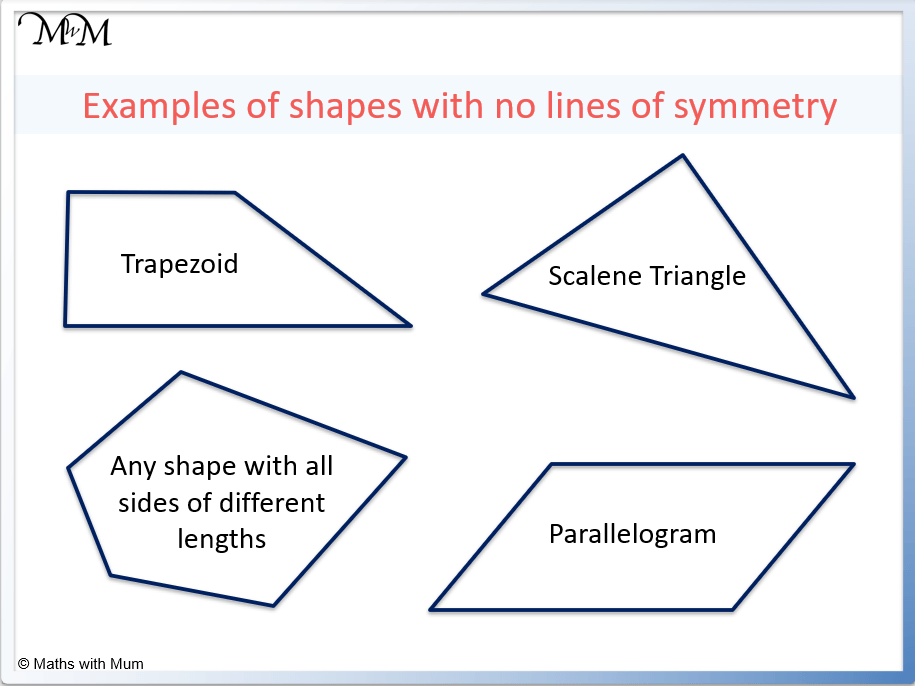#### Lines of Symmetry of Triangles

Equilateral triangles have 3 lines of symmetry, which each pass through each corner to the middle of the opposite side. Isosceles triangles have 1 line of symmetry, which is directly between the two equal sides and equal angles. Scalene triangles have no equal sides and so, they have no lines of symmetry.

Type of Triangle Number of Lines of Symmetry
Scalene Triangle 0
Isosceles Triangle 1
Equilateral Triangle 3#### Lines of Symmetry of a Square

A square has 4 lines of symmetry. There are 2 lines of symmetry passing from each corner to the opposite corner. There are a further 2 lines of symmetry passing through the middle of each side to the middle of the opposite side.#### Lines of Symmetry of a Rectangle

A rectangle has 2 lines of symmetry. These lines pass from the middle of each side to the middle of the opposite side. There are no lines of symmetry passing through the diagonals of the rectangle.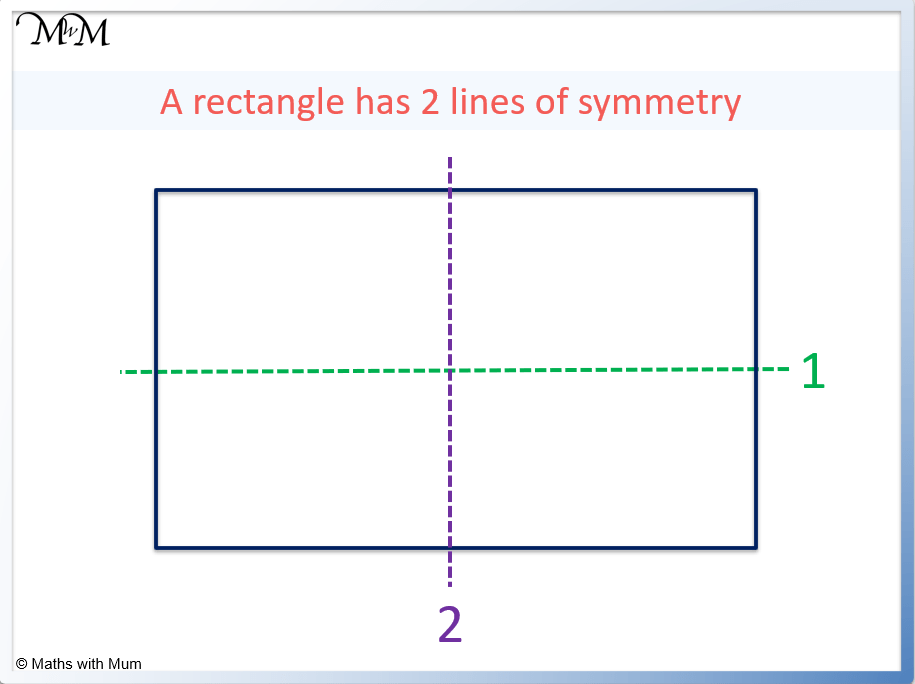The diagonals of a rectangle are not lines of symmetry.We can see that the diagonals of a rectangle are not lines of symmetry.

#### Lines of Symmetry of a Rhombus

A rhombus has 2 lines of symmetry. These lines of symmetry pass through the diagonals of the rhombus, from each corner to the opposite corner.

Here are the 2 lines of symmetry of a rhombus.#### Lines of Symmetry of a Parallelogram

A parallelogram has 0 lines of symmetry. This is because the diagonals of a parallelogram are not symmetrical. If we fold a parallelogram along its diagonals, it will not fold exactly in half without overlap.

#### Lines of Symmetry of a Kite

Every kite has one line of symmetry. This line of symmetry passes vertically down the centre of the kite.#### Lines of Symmetry on a Trapezium

Trapeziums have no lines of symmetry unless they are isosceles trapeziums which have 1 line of symmetry. This means that a trapezium only has a line of symmetry if both of its diagonal sides are the same length. In this case, the line of symmetry passes directly between the 2 diagonal sides.#### Lines of Symmetry of a Pentagon

A regular pentagon has 5 lines of symmetry. Each line of symmetry passes from each of the 5 corners, through the centre of the pentagon to the middle of the opposite side.

Here are the 5 lines of symmetry shown on a regular pentagon.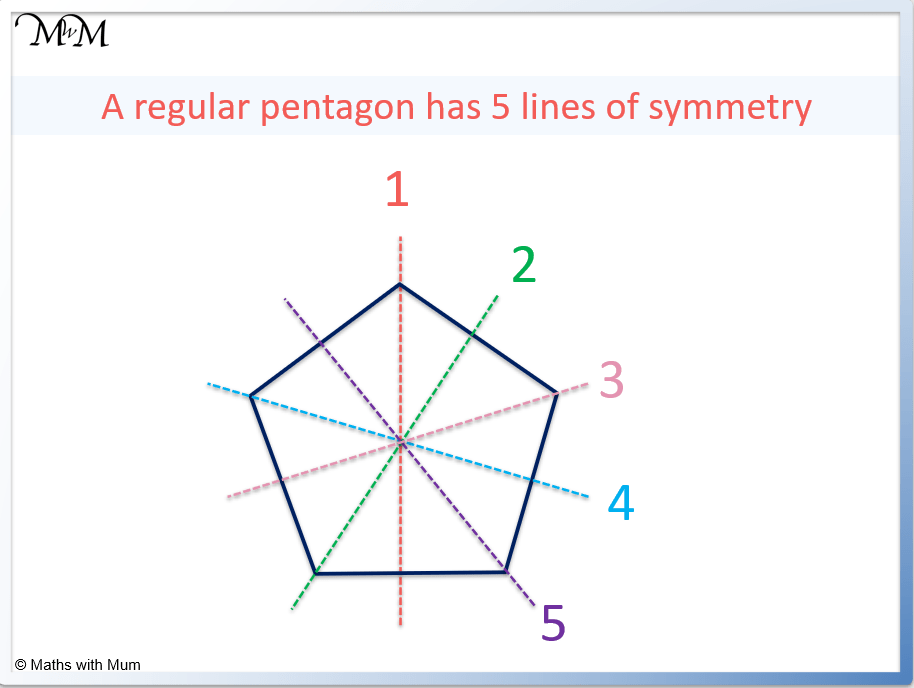#### Lines of Symmetry of a Hexagon

A regular hexagon has 6 lines of symmetry. 3 lines of symmetry pass from each of the corners to the opposite corner. The other 3 lines of symmetry pass from the middle of each side to the middle of the opposite side.

Here are the lines of symmetry of a regular hexagon.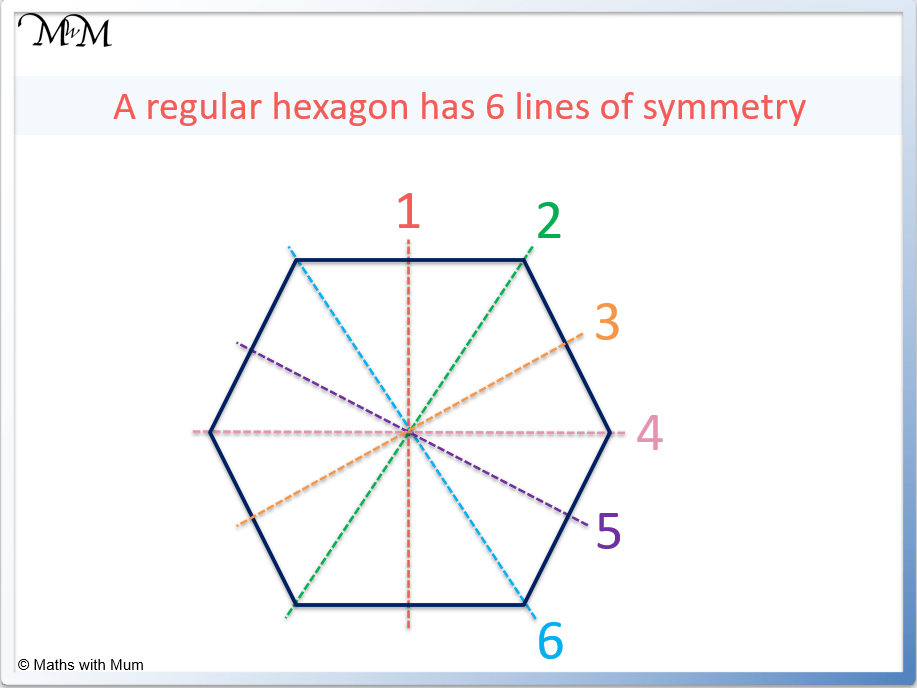#### Lines of Symmetry on an Octagon

A regular octagon has 8 lines of symmetry. 4 lines of symmetry pass from each of the corners to the opposite corner. The other 4 lines of symmetry pass from the middle of each side to the middle of the opposite side.

Here are the lines of symmetry on a regular octagon.#### Lines of Symmetry of a Circle

A circle has infinite lines of symmetry. This is because the circle looks the same in any direction. Each line of symmetry is the diameter of the circle, passing from one side to the other through the centre. There are infinite diameter lines that can be drawn and so, there are infinite lines of symmetry on a circle.

Here are just some of the lines of symmetry of a circle.## Letters with Lines of Symmetry

• The letters of F, G, J, L, N, P, Q, R, S and Z do not have any lines of symmetry.
• The letters of A, B, C, D, E, K, M, T, U, V, W and Y have 1 line of symmetry.
• The letters of H, I, O and X have 2 lines of symmetry.

Here are the capital letters of the alphabet on a worksheet. Draw the lines of symmetry on these capital letters.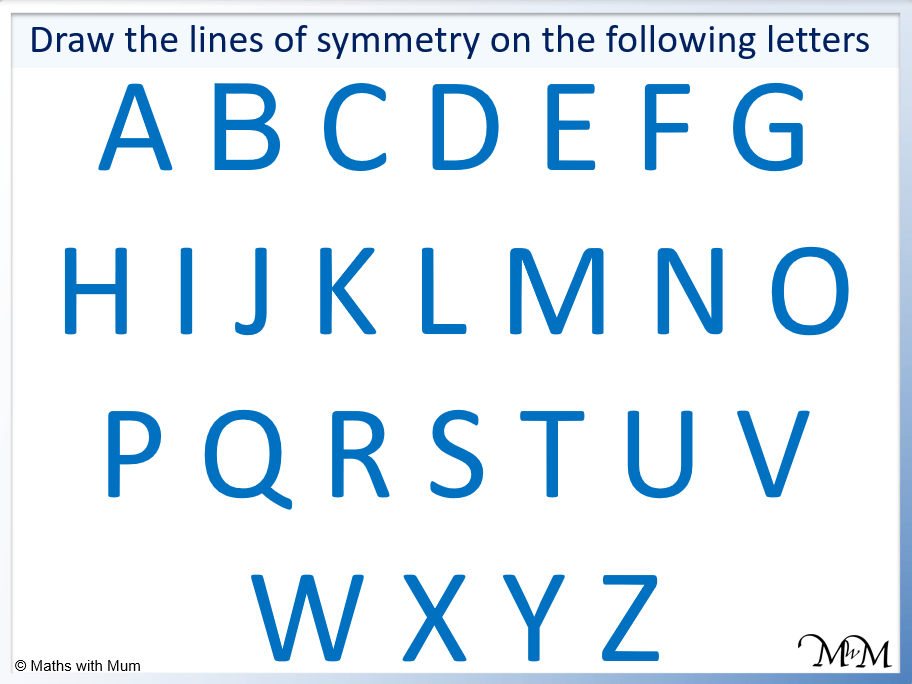Here are the answers to this worksheet with the lines of symmetry of the letters shown.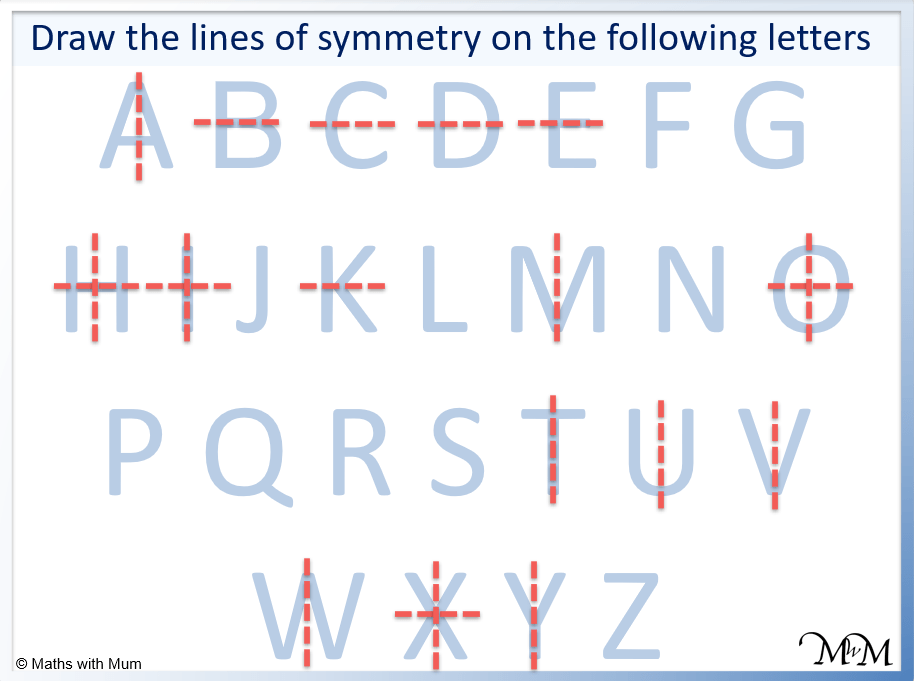Now try our lesson on Rotational Symmetry where we learn how to find the order of rotational symmetry for a shape.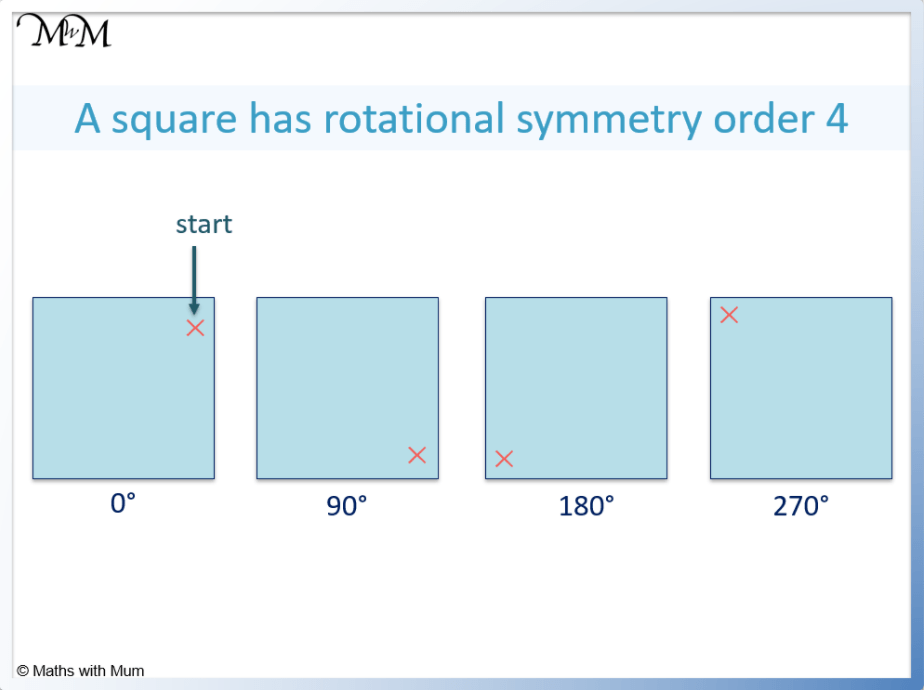error: Content is protected !!